Intťgration numťrique

Intťgration numťrique

Ce document prťsente quelques mťthodes classiques de calcul numťrique d'intťgrales. Il est destinť ŗ des ťtudiants de licence.

Vous trouverez ici le fichier pdf de ce document docintegnum.pdf

I Introduction

Intťgration numťrique → I Introduction

I-1 ProblŤme ťtudiť

Intťgration numťriqueI Introduction → I-1 ProblŤme ťtudiť
Soit une fonction $f:\left[a,\phantom{\rule{thickmathspace}{0ex}}b\right]⟶ℝ$ intťgrable. Nous nous intťressons au calcul de son intťgrale sur $\left[a,\phantom{\rule{thickmathspace}{0ex}}b\right]$:
$I\left(f\right)={\begin{array}{c}\int \end{array}}_{a}^{b}f\left(x\right)\phantom{\rule{thickmathspace}{0ex}}\mathrm{dx}.$
Dans ce chapitre on prťsente la thťorie des quelques mťthodes classiques de calcul numťrique de $I\left(f\right)$. Ces mťthodes sont appelťes mťthodes de quadrature . Pour chaque mťthode, on s'intťresse ŗ son ordre, ŗ l'ťtude de sa convergence et ŗ l'ťtude de son erreur de convergence. On dťveloppe aussi quelques idťes nťcessaires ŗ l'ťcriture d'un programme numťrique pour le calcul de $I\left(f\right)$.

I-2 Notations et dťfinitions

Intťgration numťriqueI Introduction → I-2 Notations et dťfinitions
Soit $\phantom{\rule{thickmathspace}{0ex}}f:\left[a,\phantom{\rule{thickmathspace}{0ex}}b\right]⟶ℝ\phantom{\rule{thickmathspace}{0ex}}$ bornťe et soit
$\sigma =\left\{a={x}_{0}<{x}_{1}<\cdots <{x}_{n}=b\right\}\phantom{\rule{thickmathspace}{0ex}}$
une subdivision de $\phantom{\rule{thickmathspace}{0ex}}\left[a,\phantom{\rule{thickmathspace}{0ex}}b\right]\phantom{\rule{thickmathspace}{0ex}}$ de pas
$\phantom{\rule{thickmathspace}{0ex}}\mid \sigma \mid =\underset{0\le i\le n}{\mathrm{sup}}\mid {x}_{i+1}-{x}_{i}\mid$
On pose:
$\phantom{\rule{thickmathspace}{0ex}}{m}_{i}=\underset{x\in \right]{x}_{i},\phantom{\rule{thickmathspace}{0ex}}\phantom{\rule{thickmathspace}{0ex}}{x}_{i+1}\left[}{\mathrm{inf}}f\left(x\right),\phantom{\rule{1em}{0ex}}{M}_{i}=\underset{x\in \right]{x}_{i},\phantom{\rule{thickmathspace}{0ex}}{x}_{i+1}\left[}{\mathrm{sup}}f\left(x\right)$
${s}_{\sigma }\left(f\right)=\sum _{i=0}^{n-1}\left({x}_{i+1}-{x}_{i}\right){m}_{i},\phantom{\rule{1em}{0ex}}{S}_{\sigma }\left(f\right)=\sum _{i=0}^{n-1}\left({x}_{i+1}-{x}_{i}\right){M}_{i}$
${I}_{+}\left(f\right)=\underset{\sigma }{\mathrm{inf}}{S}_{\sigma }\left(f\right),\phantom{\rule{1em}{0ex}}{I}_{-}\left(f\right)=\underset{\sigma }{\mathrm{sup}}{s}_{\sigma }\left(f\right)$

Dťfinition [Intťgrale de Riemann]

La fonction $f$ est dite Riemann intťgrable si ${I}_{+}\left(f\right)={I}_{-}\left(f\right)$. Dans ce cas, on note $I\left(f\right)={\begin{array}{c}\int \end{array}}_{a}^{b}f\left(x\right)\phantom{\rule{thickmathspace}{0ex}}\mathrm{dx}$ le rťel ${I}_{+}\left(f\right)={I}_{-}\left(f\right)$ et on l'appelle l'intťgrale de Riemann associťe ŗ $f$.

Remarque

1. Toute fonction continue par morceaux est Riemann intťgrable.
2. Toute fonction monotone est Riemann intťgrable.

I-3 Rťsultats fondamentaux

Intťgration numťriqueI Introduction → I-3 Rťsultats fondamentaux

Proposition

Si $f:\left[a,\phantom{\rule{thickmathspace}{0ex}}b\right]⟶ℝ$ est Riemann intťgrable, alors
${\begin{array}{c}\int \end{array}}_{a}^{b}f\left(x\right)\phantom{\rule{thickmathspace}{0ex}}\mathrm{dx}=\underset{\mid \sigma \mid ⟶0}{\mathrm{lim}}{S}_{\sigma }\left(f\right)=\underset{\mid \sigma \mid ⟶0}{\mathrm{lim}}{s}_{\sigma }\left(f\right)$
ou d'une maniŤre ťquivalente
$\forall \epsilon >0,\phantom{\rule{thickmathspace}{0ex}}\exists \eta >0\phantom{\rule{thickmathspace}{0ex}}\text{tq}\phantom{\rule{thickmathspace}{0ex}}\forall \sigma ,\phantom{\rule{thickmathspace}{0ex}}\mid \sigma \mid <\eta ⟹\begin{array}{ccc}\mid I\left(f\right)-{S}_{\sigma }\left(f\right)\mid & \le & \epsilon \\ \mid I\left(f\right)-{s}_{\sigma }\left(f\right)\mid & \le & \epsilon \end{array}$

Remarque

Si $f:\left[a,\phantom{\rule{thickmathspace}{0ex}}b\right]⟶ℝ\phantom{\rule{thickmathspace}{0ex}}$ est continue alors
$\phantom{\rule{thickmathspace}{0ex}}\underset{x\in \right]{x}_{i},\phantom{\rule{thickmathspace}{0ex}}{x}_{i+1}\left[}{\mathrm{inf}}f\left(x\right)=\underset{x\in \left[{x}_{i},\phantom{\rule{thickmathspace}{0ex}}{x}_{i+1}\right]}{\mathrm{inf}}f\left(x\right)\phantom{\rule{thickmathspace}{0ex}}\text{et}\phantom{\rule{thickmathspace}{0ex}}\underset{x\in \right]{x}_{i},\phantom{\rule{thickmathspace}{0ex}}{x}_{i+1}\left[}{\mathrm{sup}}f\left(x\right)=\underset{x\in \left[{x}_{i},\phantom{\rule{thickmathspace}{0ex}}{x}_{i+1}\right]}{\mathrm{sup}}f\left(x\right)$

ThťorŤme

Si $f:\left[a,\phantom{\rule{thickmathspace}{0ex}}b\right]⟶ℝ\phantom{\rule{thickmathspace}{0ex}}$ est continue alors
$\phantom{\rule{thickmathspace}{0ex}}{\begin{array}{c}\int \end{array}}_{a}^{b}f\left(x\right)\phantom{\rule{thickmathspace}{0ex}}\mathrm{dx}=\underset{n⟶+\infty }{\mathrm{lim}}\frac{b-a}{n}\sum _{i=0}^{n-1}{m}_{i}=\underset{n⟶+\infty }{\mathrm{lim}}\frac{b-a}{n}\sum _{i=0}^{n-1}{M}_{i}$
et d'une faÁon plus gťnťrale
${\begin{array}{c}\int \end{array}}_{a}^{b}f\left(x\right)\phantom{\rule{thickmathspace}{0ex}}\mathrm{dx}=\underset{n⟶+\infty }{\mathrm{lim}}\frac{b-a}{n}\sum _{i=0}^{n-1}f\left({c}_{i}\right)\phantom{\rule{thickmathspace}{0ex}}\text{avec}\phantom{\rule{thickmathspace}{0ex}}{c}_{i}\in \left[{x}_{i},\phantom{\rule{thickmathspace}{0ex}}{x}_{i+1}\right]$

II Formules de quadrature et leur ordre

Intťgration numťrique → II Formules de quadrature et leur ordre

II-1 Idťe de base

La plupart des algorithmes numťriques procŤdent comme suit : on subdivise l'intervalle $\left[a,\phantom{\rule{thickmathspace}{0ex}}b\right]$ en plusieurs sous-intervalles $\sigma =\left\{a={x}_{0}<{x}_{1}<\cdots <{x}_{n}=b\right\}$ et on utilise le fait que
${\begin{array}{c}\int \end{array}}_{a}^{b}f\left(x\right)\phantom{\rule{thickmathspace}{0ex}}\mathrm{dx}=\sum _{i=0}^{n-1}{\begin{array}{c}\int \end{array}}_{{x}_{i}}^{{x}_{i+1}}f\left(x\right)\phantom{\rule{thickmathspace}{0ex}}\mathrm{dx}$
De cette maniŤre, on est amenť au calcul de plusieurs intťgrales pour lesquelles la longueur de l'intervalle d'intťgration est relativement petite. Prenons une de ces intťgrales, notons ${h}_{i}={x}_{i+1}-{x}_{i}$ la longueur de l'intervalle et $g\left(t\right)=f\left({x}_{i}+t{h}_{i}\right)$. Un changement de variable nous donne alors:
$\phantom{\rule{thickmathspace}{0ex}}{\begin{array}{c}\int \end{array}}_{{x}_{i}}^{{x}_{i+1}}f\left(x\right)\phantom{\rule{thickmathspace}{0ex}}\mathrm{dx}={h}_{i}{\begin{array}{c}\int \end{array}}_{0}^{1}g\left(t\right)\phantom{\rule{thickmathspace}{0ex}}\mathrm{dt}$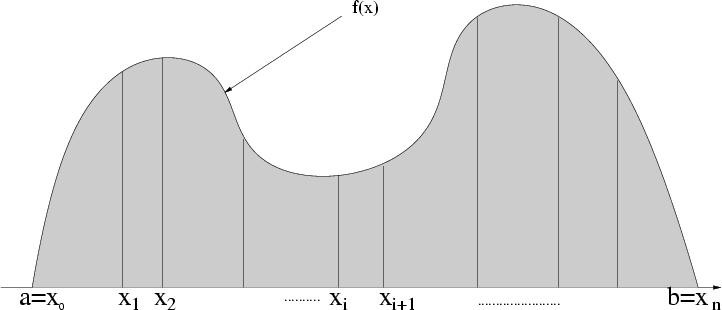Il reste alors ŗ calculer une approximation de
${\begin{array}{c}\int \end{array}}_{0}^{1}g\left(t\right)\mathrm{dt}$

II-2 Mťthode des rectangles ŗ gauche

Intťgration numťriqueII Formules de quadrature et leur ordre → II-2 Mťthode des rectangles ŗ gauche
${\begin{array}{c}\int \end{array}}_{0}^{1}g\left(t\right)\mathrm{dt}\approx g\left(0\right)$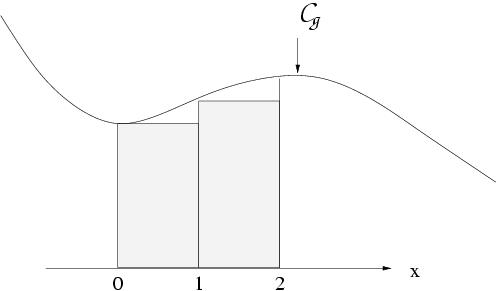L'aire du domaine limitť par les droites $x=0,\phantom{\rule{thickmathspace}{0ex}}x=1$, l'axe $\mathrm{Ox}$ et ${𝒞}_{g}$ est approchťe par l'aire du rectangle de base $\left[0,\phantom{\rule{thickmathspace}{0ex}}1\right]$
Exercice
Mťthode du rectangle

II-3 Mťthode des rectangles ŗ droite

Intťgration numťriqueII Formules de quadrature et leur ordre → II-3 Mťthode des rectangles ŗ droite
${\begin{array}{c}\int \end{array}}_{0}^{1}g\left(t\right)\mathrm{dt}\approx g\left(1\right)$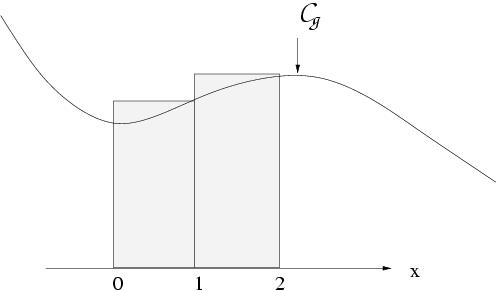L'aire du domaine limitť par les droites $x=0,\phantom{\rule{thickmathspace}{0ex}}x=1$, l'axe $\mathrm{Ox}$ et ${𝒞}_{g}$ est approchťe par l'aire du rectangle de base $\left[0,\phantom{\rule{thickmathspace}{0ex}}1\right]$

II-4 Mťthode du point milieu

${\begin{array}{c}\int \end{array}}_{0}^{1}g\left(t\right)\mathrm{dt}\approx g\left(1/2\right)$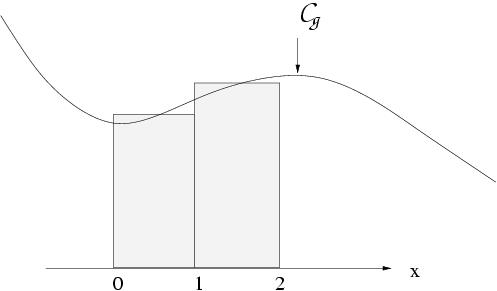L'aire du domaine limitť par les droites $x=0,\phantom{\rule{thickmathspace}{0ex}}x=1$, l'axe $\mathrm{Ox}$ et ${𝒞}_{g}$ est approchťe par l'aire du rectangle de base $\left[0,\phantom{\rule{thickmathspace}{0ex}}1\right]$
Exercice
Mťthode du point milieu

II-5 Mťthode du trapŤze

${\begin{array}{c}\int \end{array}}_{0}^{1}g\left(t\right)\mathrm{dt}\approx 1/2\left(g\left(0\right)+g\left(1\right)\right)$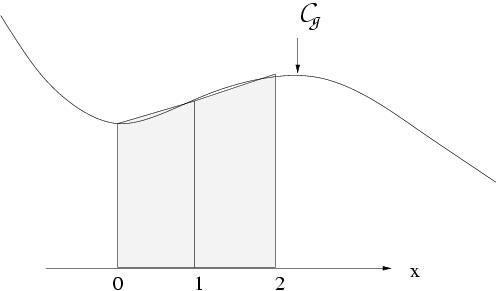L'aire du domaine limitť par les droites $x=0,\phantom{\rule{thickmathspace}{0ex}}x=1$, l'axe $\mathrm{Ox}$ et ${𝒞}_{g}$ est approchťe par l'aire du trapŤze de base $\left[0,\phantom{\rule{thickmathspace}{0ex}}1\right]$

II-6 Mťthode de Simpson

TraÁons la parabole passant par les trois points $\left(0,\phantom{\rule{thickmathspace}{0ex}}g\left(0\right)\right),\phantom{\rule{thickmathspace}{0ex}}\left(1/2,\phantom{\rule{thickmathspace}{0ex}}g\left(1/2\right)\right),\phantom{\rule{thickmathspace}{0ex}}\left(1,\phantom{\rule{thickmathspace}{0ex}}g\left(1\right)\right)$. En approchant l'intťgrale par l'aire sous la parabole, on obtient la formule de Simpson :
${\begin{array}{c}\int \end{array}}_{0}^{1}g\left(t\right)\mathrm{dt}\approx 1/6\left(g\left(0\right)+4g\left(1/2\right)+g\left(1\right)\right)$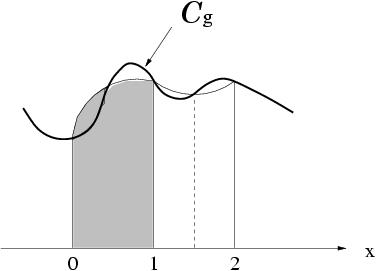L'aire du domaine limitť par les droites $x=0,\phantom{\rule{thickmathspace}{0ex}}x=1$, l'axe $\mathrm{Ox}$ et ${𝒞}_{g}$ est approchťe par l'aire grisťe.

II-7 Mťthode de Newton-Cotes

On peut gťnťraliser la mťthode de Simpson en approchant l'intťgrale par l'aire sous un polynŰme de degrť $s-1$ passant par les $s$ points ťquidistants
$\left(\left(\frac{i}{s-1},\phantom{\rule{thickmathspace}{0ex}}g\left(\frac{i}{s-1}\right)\right),\phantom{\rule{thickmathspace}{0ex}}i=0,\cdots ,s-1\right),$
on obtient des formules de quadrature appelťes formules de Newton-Cotes donnťes par la dťfinition.

Dťfinition

Une formule de quadrature est dite de Newton-Cotes ŗ $s$ ťtages si elle est de la forme:
${\begin{array}{c}\int \end{array}}_{0}^{1}g\left(t\right)\mathrm{dt}\approx \sum _{i=1}^{s}{b}_{i}g\left({c}_{i}\right).$
Les ${c}_{i}$ sont les noeuds de la formule de quadrature et les ${b}_{i}$ sont les poids .

Dťfinition

On dit que l'ordre ordre d'une formule de quadrature de la formule de quadrature est $p$ si elle est exacte pour tous les polynŰmes de degrť infťrieur ou ťgal ŗ $p-1$, c'est-ŗ-dire: pour $g$ polynŰme de degrť $\le p-1$,
${\begin{array}{c}\int \end{array}}_{0}^{1}g\left(t\right)\mathrm{dt}=\sum _{i=1}^{s}{b}_{i}g\left({c}_{i}\right)$

On voit que les formules du point milieu et des trapŤzes sont d'ordre 2. La formule de Newton-Cotes ŗ $s$ ťtages a un ordre $p$ supťrieur ou ťgal ŗ $s$.
Le tableau suivant rťsume l'ordre ainsi que les poids des diffťrentes mťthodes de quadrature pour $s\le 7.$
Tableau
 s ordre ${b}_{1}$ ${b}_{2}$ ${b}_{3}$ ${b}_{4}$ ${b}_{5}$ ${b}_{6}$ ${b}_{7}$ nom 1 1 1 rectangle 1 2 1 pt. milieu 2 2 $\frac{1}{2}$ $\frac{1}{2}$ trapŤze 3 4 $\frac{1}{6}$ $\frac{4}{6}$ $\frac{1}{6}$ Simpson 4 4 $\frac{1}{8}$ $\frac{3}{8}$ $\frac{3}{8}$ $\frac{1}{8}$ Newton 5 6 $\frac{7}{90}$ $\frac{32}{90}$ $\frac{12}{90}$ $\frac{32}{90}$ $\frac{7}{90}$ Boole 6 6 $\frac{19}{288}$ $\frac{75}{288}$ $\frac{50}{288}$ $\frac{50}{288}$ $\frac{75}{288}$ $\frac{19}{288}$ Boole 7 8 $\frac{41}{840}$ $\frac{216}{840}$ $\frac{27}{840}$ $\frac{272}{840}$ $\frac{27}{840}$ $\frac{216}{840}$ $\frac{41}{840}$ Weddle

Exercice

II-8-2 Condition nťcessaire et suffisante

Intťgration numťriqueII Formules de quadrature et leur ordreII-8 Ordre → II-8-2 Condition nťcessaire et suffisante

ThťorŤme

La formule de quadrature est d'ordre $p$ si et seulement si:
$\sum _{i=1}^{s}{b}_{i}{c}_{i}^{q-1}=\frac{1}{q},\phantom{\rule{thickmathspace}{0ex}}\text{pour}q=1,\phantom{\rule{thickmathspace}{0ex}}\cdots ,\phantom{\rule{thickmathspace}{0ex}}p$

Preuve
La nťcessitť de l'ťquivalence ) est une consťquence de la formule ) si l'on pose $g\left(t\right)={t}^{q-1}$. Pour en montrer la suffisance, on utilise le fait qu'un polynŰme de degrť $p-1$ est une combinaison linťaire de $1,\phantom{\rule{thickmathspace}{0ex}}t,\phantom{\rule{thickmathspace}{0ex}}\cdots ,\phantom{\rule{thickmathspace}{0ex}}{t}^{p-1}$ et que l'intťgrale ${\begin{array}{c}\int \end{array}}_{0}^{1}g\left(t\right)\phantom{\rule{thickmathspace}{0ex}}\mathrm{dt}$ ainsi que l'expression $\sum _{i=1}^{s}{b}_{i}g\left({c}_{i}\right)$ sont linťaires en $g$.

Remarque

1. En fixant les noeuds ${c}_{1},\phantom{\rule{thickmathspace}{0ex}}{c}_{2},\phantom{\rule{thickmathspace}{0ex}}\cdots ,\phantom{\rule{thickmathspace}{0ex}}{c}_{s}$ (distincts), la condition avec $p=s$ reprťsente un systŤme linťaire pour ${b}_{1},\phantom{\rule{thickmathspace}{0ex}}{b}_{2},\phantom{\rule{thickmathspace}{0ex}}\cdots ,\phantom{\rule{thickmathspace}{0ex}}{b}_{s}$
$\left(\begin{array}{cccc}1& 1& \cdots & 1\\ & & & \\ {c}_{1}& {c}_{2}& \cdots & {c}_{s}\\ & & & \\ ⋮& ⋮& & ⋮\\ & & & \\ {c}_{1}^{s-1}& {c}_{2}^{s-1}& \cdots & {c}_{s}^{s-1}\\ \end{array}\right)\left(\begin{array}{c}{b}_{1}\\ {b}_{2}\\ ⋮\\ {b}_{s}\\ \end{array}\right)=\left(\begin{array}{c}1\\ \frac{1}{2}\\ ⋮\\ \frac{1}{s}\\ \end{array}\right)$

Comme la matrice dans la formule est inversible (matrice de Vandermonde), la rťsolution de ce systŤme nous donne une formule de quadrature d'ordre $p=s$.
2. Si l'on vťrifie les conditions pour la formule de Simpson, on fait une observation intťressante: par dťfinition, il est ťvident que la condition est satisfaite pour $q=1$, $2$, $3$, mais on remarque qu'elle est aussi satisfaite pour $q=4$. En effet:
$\begin{array}{c}\frac{1}{6}{0}^{3}+\frac{4}{6}{\left(\frac{1}{2}\right)}^{3}+\frac{1}{6}{1}^{3}=\frac{1}{4}\\ \frac{1}{6}{0}^{4}+\frac{4}{6}{\left(\frac{1}{2}\right)}^{4}+\frac{1}{6}{1}^{4}=\frac{5}{24}\ne \frac{1}{5}.\end{array}$
Elle est donc d'ordre 4. Par consťquent, elle n'est pas seulement exacte pour les polynŰmes de degrť 2 mais aussi pour les polynŰmes de degrť 3. Ceci est est une propriťtť qui peut Ítre gťnťralisťe aux formules de quadrature symťtriques (c'est-ŗ-dire ${c}_{i}=1-{c}_{s+1-i},\phantom{\rule{thickmathspace}{0ex}}{b}_{i}={b}_{s+1-i},\phantom{\rule{thickmathspace}{0ex}}\forall \phantom{\rule{thickmathspace}{0ex}}1\le i\le s$).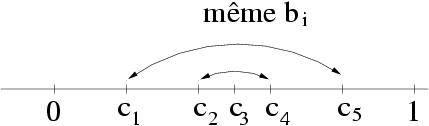Coefficients et noeuds d'une formule de quadrature symťtrique

ThťorŤme

Une formule de quadrature symťtrique a toujours un ordre pair: si elle est exacte pour les polynŰmes de degrť $\le \phantom{\rule{thickmathspace}{0ex}}2m-2$, elle est exacte pour les polynŰmes de degrť $\le \phantom{\rule{thickmathspace}{0ex}}2m-1$.

Preuve
Chaque polynŰme $g\left(t\right)$ de degrť $\le \phantom{\rule{thickmathspace}{0ex}}2m-1$ peut Ítre ťcrit sous la forme
$g\left(t\right)=c\left(t-\frac{1}{2}{\right)}^{2m-1}+h\left(t\right)$
$h\left(t\right)$ est un polynŰme de degrť $\le \phantom{\rule{thickmathspace}{0ex}}2m-2$ et oý $c$ est une constante. Il suffit alors de montrer que la formule symťtrique est exacte pour $\left(t-\frac{1}{2}{\right)}^{2m-1}$. A cause de la symťtrie de cette fonction, la valeur exacte vaut
${\begin{array}{c}\int \end{array}}_{0}^{1}\left(t-\frac{1}{2}{\right)}^{2m-1}\mathrm{dt}=0$
Pour une formule de quadrature symťtrique on a
${b}_{i}\left({c}_{i}-\frac{1}{2}{\right)}^{2m-1}+{b}_{s+1-i}\left({c}_{s+1-i}-\frac{1}{2}{\right)}^{2m-1}=0$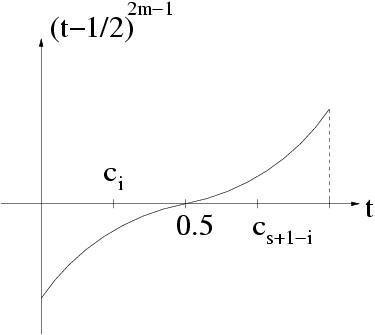Donc l'approximation numťrique de ${\begin{array}{c}\int \end{array}}_{0}^{1}\left(t-\frac{1}{2}{\right)}^{2m-1}\mathrm{dt}$ est ťgalement nulle.

III Mise en oeuvre sur Matlab

Intťgration numťrique → III Mise en oeuvre sur Matlab

III-1 Notations

Ici nous allons exťcuter sur Matlab quelques mťthodes de quadrature classiques pour approcher la valeur de l'intťgrale
${I}_{\mathrm{exa}}={\begin{array}{c}\int \end{array}}_{1}^{2}\frac{1}{t}\phantom{\rule{thickmathspace}{0ex}}\mathrm{dt}=\mathrm{log}\left(2\right)$
avec une subdivision de l'intervalle $\left[1,\phantom{\rule{thickmathspace}{0ex}}2\right]$ correspondante ŗ
${\alpha }_{i}=1+\frac{1}{N}i;\phantom{\rule{thickmathspace}{0ex}}0\le i\le N\text{et}N=4.$

III-2 Mťthode des rectangles ŗ gauche

Intťgration numťriqueIII Mise en oeuvre sur Matlab → III-2 Mťthode des rectangles ŗ gauche
On note ${I}_{\mathrm{rg}}$ l'approximation de ${I}_{\mathrm{exa}}$ par la mťthode des rectangles ŗ gauche et ${E}_{\mathrm{rg}}$ l'erreur commise. Voici un programme Matlab qui calcule ${I}_{\mathrm{rg}}$ et ${E}_{\mathrm{rg}}$:
Code Matlab
```%%%%%%%%%%%%%%%%%%%%%%%%
%      Donnees
%%%%%%%%%%%%%%%%%%%%%%%%
Iexa = log(2);
alpha = 1;
beta = 2;
N = 4;
h = (beta - alpha)/N;
x = [alpha:h:beta];
f = inline('1/x','x');
%%%%%%%%%%%%%%%%%%%%%%%%
% Corps du programme
%%%%%%%%%%%%%%%%%%%%%%%%
Irg = 0.0;
for i = 1:N
Irg = Irg + h*f(x(i));
end
%%%%%%%%%%%%%%%%%%%%%%%%%%
% Affichage des resultats
%%%%%%%%%%%%%%%%%%%%%%%%%%
Irg
Erg = abs(Iexa - Irg)
%%%%%%%%%%%%%%%%%%%%%%%%
```

Les rťsultats obtenus par ce programme sont:
```Irg = 7.595238095e-01
Erg = 6.637662896e-02
```

III-3 Mťthode des trapŤzes

Intťgration numťriqueIII Mise en oeuvre sur Matlab → III-3 Mťthode des trapŤzes
On note ${I}_{\mathrm{tr}}$ l'approximation de ${I}_{\mathrm{exa}}$ par la mťthode des trapŤzes et ${E}_{\mathrm{tr}}$ l'erreur commise. Voici un programme Matlab qui calcule ${I}_{\mathrm{tr}}$ et ${E}_{\mathrm{tr}}$:
Code Matlab
```%%%%%%%%%%%%%%%%%%%%%%%%
%      Donnees
%%%%%%%%%%%%%%%%%%%%%%%%
Iexa = log(2);
alpha = 1;
beta = 2;
N = 4;
h = (beta - alpha)/N;
x = [alpha:h:beta];
f = inline('1/x','x');
%%%%%%%%%%%%%%%%%%%%%%%%
% Corps du programme
%%%%%%%%%%%%%%%%%%%%%%%%
Itr = 0.0;
for i = 1:N
Itr = Itr + h*(0.5*f(x(i)) + 0.5*f(x(i+1)));
end
%%%%%%%%%%%%%%%%%%%%%%%%%%
% Affichage des resultats
%%%%%%%%%%%%%%%%%%%%%%%%%%
Itr
Etr = abs(Iexa - Itr)
```

Les rťsultats obtenus par ce programme sont:
```Itr = 6.970238095e-01
Etr = 3.876628964e-03
```

III-4 Mťthode de Simpson

Intťgration numťriqueIII Mise en oeuvre sur Matlab → III-4 Mťthode de Simpson
On note ${I}_{\mathrm{si}}$ l'approximation de ${I}_{\mathrm{exa}}$ par la mťthode de Simpson et ${E}_{\mathrm{si}}$ l'erreur commise. Voici un programme Matlab qui calcule ${I}_{\mathrm{si}}$ et ${E}_{\mathrm{si}}$:
Code Matlab
```%%%%%%%%%%%%%%%%%%%%%%%%
%      Donnees
%%%%%%%%%%%%%%%%%%%%%%%%
Iexa = log(2);
alpha = 1;
beta = 2;
N = 4;
h = (beta - alpha)/N;
x = [alpha:h:beta];
f = inline('1/x','x');
%%%%%%%%%%%%%%%%%%%%%%%%
% Corps du programme
%%%%%%%%%%%%%%%%%%%%%%%%
Isi = 0.0;
for i = 1:N
Isi = Isi+h*(1/6*f(x(i))+2/3*f((x(i)+x(i+1))/2)+1/6*f(x(i+1)));
end
%%%%%%%%%%%%%%%%%%%%%%%%%%
% Affichage des resultats
%%%%%%%%%%%%%%%%%%%%%%%%%%
Isi
Es = abs(Iexa - Isi)
```

Les rťsultats obtenus par ce programme sont:
```Isi = 6.931545307e-01
Esi = 7.350094585e-06
```

III-5 Commentaires des rťsultats

Intťgration numťriqueIII Mise en oeuvre sur Matlab → III-5 Commentaires des rťsultats
On voit bien que l'erreur absolue obtenue par la mťthode de Simpson est beaucoup plus faible que celles obtenues par les deux autres. Ceci confirme la rŤgle: plus l'ordre de la mťthode est grand, plus la prťcision est bonne .

IV Etude de l'erreur d'une mťthode de quadrature

Intťgration numťrique → IV Etude de l'erreur d'une mťthode de quadrature
Pour ťtudier l'erreur commise en approchant une intťgrale par l'une des formules de quadrature, nous commenÁons par une expťrience numťrique :

IV-1-1 Nombre d'ťvaluation

Prenons une fonction $f$ dťfinie sur $\left[a,\phantom{\rule{thickmathspace}{0ex}}b\right]$, subdivisons l'intervalle en plusieurs sous-intervalles ťquidistants ( $h=\frac{b-a}{N}$) et appliquons l'une des formules de quadrature du paragraphe prťcťdent. Ensuite, ťtudions l'erreur (en ťchelle logarithmique),
$\begin{array}{ccc}E\left(f\right)& =& {\begin{array}{c}\int \end{array}}_{a}^{b}f\left(x\right)\phantom{\rule{thickmathspace}{0ex}}\mathrm{dx}-\sum _{j=0}^{N-1}{\begin{array}{c}\int \end{array}}_{{x}_{j}}^{{x}_{j+1}}f\left(x\right)\phantom{\rule{thickmathspace}{0ex}}\mathrm{dx}\\ & & \\ & =& {\begin{array}{c}\int \end{array}}_{a}^{b}f\left(x\right)\phantom{\rule{thickmathspace}{0ex}}\mathrm{dx}-\sum _{j=0}^{N-1}h{\begin{array}{c}\int \end{array}}_{0}^{1}f\left({x}_{j}+th\right)\phantom{\rule{thickmathspace}{0ex}}\mathrm{dt}\\ & & \\ & =& {\begin{array}{c}\int \end{array}}_{a}^{b}f\left(x\right)\phantom{\rule{thickmathspace}{0ex}}\mathrm{dx}-\sum _{j=0}^{N-1}h\sum _{i=1}^{s}{b}_{i}f\left({x}_{j}+{c}_{i}h\right),\end{array}$
en fonction du nombre d'ťvaluations ${f}_{e}$ de la fonction $f$ pour Newton-Cotes : ${f}_{e}$ est dťfini par:
${f}_{e}=N\left(s-1\right)+1$
Le nombre ${f}_{e}$ repťsente une mesure pour le travail effectuť par l'ordinateur.

IV-1-2 Exemple

Voici les rťsultats obtenus par les formules de Newton-Cotes (trapŤzes, Simpson, Boole) pour l'int'grale
${I}_{\mathrm{exa}}=\mathrm{sin}\left(2\right)={\begin{array}{c}\int \end{array}}_{0}^{2}\mathrm{cos}\left(x\right)\phantom{\rule{thickmathspace}{0ex}}\mathrm{dx}$
et $N=1,\phantom{\rule{thickmathspace}{0ex}}2,\phantom{\rule{thickmathspace}{0ex}}4,\phantom{\rule{thickmathspace}{0ex}}8,\phantom{\rule{thickmathspace}{0ex}}16,\phantom{\rule{thickmathspace}{0ex}}\cdots$
Code Matlab
```clear all;
Iexa = sin(2);
alpha = 0;
beta = 2;
f = inline('cos(x)','x');
%--------------------------
%--------------------------
% Mťthode des trapŤzes
%--------------------------
%--------------------------
s = 2;
for j = 1:1:10
%%%%%%%%%%%%%%%%%%%%%%%%
%      Donnees
%%%%%%%%%%%%%%%%%%%%%%%%
N = 2^j;
fetr(j) = log10(N*(s-1) +1);
h = (beta - alpha)/N;
x = [alpha:h:beta];
%%%%%%%%%%%%%%%%%%%%%%%%
% Corps du programme
%%%%%%%%%%%%%%%%%%%%%%%%
Itr=0.0;
for i = 1:N
Itr = Itr + h*(1/2*f(x(i)) + 1/2*f(x(i+1)));
end
%%%%%%%%%%%%%%%%%%%%%%%%%%
% Affichage des resultats
%%%%%%%%%%%%%%%%%%%%%%%%%%
Etr(j) = log10(abs(Iexa - Itr)) ;
end
%--------------------------
%--------------------------
% Mťthode de Simpson
%--------------------------
%--------------------------
s = 3;
for j=1:1:10
%%%%%%%%%%%%%%%%%%%%%%%%
%      Donnees
%%%%%%%%%%%%%%%%%%%%%%%%
N = 2^j;
fesi(j) = log10(N*(s-1) +1);
h = (beta - alpha)/N;
x = [alpha:h:beta];
%%%%%%%%%%%%%%%%%%%%%%%%
% Corps du programme
%%%%%%%%%%%%%%%%%%%%%%%%
Isi = 0.0;
for i = 1:N
Isi = Isi+h*(1/6*f(x(i))+2/3*f((x(i)+x(i+1))/2)+1/6*f(x(i+1)));
end
%%%%%%%%%%%%%%%%%%%%%%%%%%
% Affichage des resultats
%%%%%%%%%%%%%%%%%%%%%%%%%%
Esi(j) = log10(abs(Iexa - Isi)) ;
end
%--------------------------
%--------------------------
% Mťthode de Boole (s=6)
%--------------------------
%--------------------------
s = 6;
for j = 1:1:8
%%%%%%%%%%%%%%%%%%%%%%%%
%      Donnees
%%%%%%%%%%%%%%%%%%%%%%%%
N = 2^j;
febo(j) = log10(N*(s-1) +1);
h = (beta - alpha)/N;
x = [alpha:h:beta];
%%%%%%%%%%%%%%%%%%%%%%%%
% Corps du programme
%%%%%%%%%%%%%%%%%%%%%%%%
Ibo = 0.0;
for i = 1:N
Ibo = Ibo + h*(19/288*f(x(i)) + 75/288*f(x(i)+h/5) +
50/288*f(x(i)+(2*h/5)) + 50/288*f(x(i)+ (3*h/5)) +
75/288*f(x(i)+ (4*h/5)) + 19/288*f(x(i+1)));
end
%%%%%%%%%%%%%%%%%%%%%%%%%%
% Affichage des resultats
%%%%%%%%%%%%%%%%%%%%%%%%%%
Ebo(j) = log10(abs(Iexa - Ibo)) ;
plot(fetr, Etr, 'k-.', fesi, Esi, 'k-+', febo, Ebo, 'k-*')
legend('TrapŤzes', 'Simpson', 'Boole (s=6)')
xlabel('log10(Erreur)');
ylabel('log10(fe)');
title('Le travail fe en fonction de l''erreur');
end
```

La figure ci-dessous montre donne les rťsultats pour $N=1,\phantom{\rule{thickmathspace}{0ex}}2,\phantom{\rule{thickmathspace}{0ex}}4,\phantom{\rule{thickmathspace}{0ex}}8,\phantom{\rule{thickmathspace}{0ex}}16.$IV-1-3 Interprťtation des rťsultats

Nous constatons que:
1. ${\mathrm{log}}_{10}\left({f}_{e}\right)$ dťpend linťairement du nombre de chiffres exacts, donnť par $-{\mathrm{log}}_{10}\left(E\left(\mathrm{cos}\right)\right)$.
2. La pente de chaque droite est $\frac{1}{p}$$p$ est l'ordre de la mťthode de quadrature.
3. Pour un travail ťquivalent (mÍme ${f}_{e}$), les formules avec un ordre ťlevť ont une meilleure prťcision.

IV-1-4 Justification des rťsultats

Etudions d'abord l'erreur faite sur un sous-intervalle de longueur $h$.
$\begin{array}{ccc}E\left(f,\phantom{\rule{thickmathspace}{0ex}}{x}_{0},\phantom{\rule{thickmathspace}{0ex}}h\right)& =& {\begin{array}{c}\int \end{array}}_{{x}_{0}}^{{x}_{0}+h}f\left(x\right)\phantom{\rule{thickmathspace}{0ex}}\mathrm{dx}-h\sum _{i=1}^{s}{b}_{i}f\left({x}_{0}+{c}_{i}h\right)\\ & & \\ & =& h\left({\begin{array}{c}\int \end{array}}_{0}^{1}f\left({x}_{0}+th\right)\phantom{\rule{thickmathspace}{0ex}}\mathrm{dt}-\sum _{i=1}^{s}{b}_{i}f\left({x}_{0}+{c}_{i}h\right)\right).\end{array}$

On considŤre la formule de quadrature d'ordre $p$. En supposant que $f$ est suffisament diffťrentiable, on peut remplacer $f\left({x}_{0}+th\right)$ et $f\left({x}_{0}+{c}_{i}h\right)$ par les sťries de Taylor au voisinage de ${x}_{0}$:
$\begin{array}{ccc}E\left(f,\phantom{\rule{thickmathspace}{0ex}}{x}_{0},\phantom{\rule{thickmathspace}{0ex}}h\right)& =& \sum _{q\ge 0}\frac{{h}^{q+1}}{q!}\left({\begin{array}{c}\int \end{array}}_{0}^{1}{t}^{q}\phantom{\rule{thickmathspace}{0ex}}\mathrm{dt}-\sum _{i=1}^{s}{b}_{i}{c}_{i}^{q}\right){f}^{\left(q\right)}\left({x}_{0}\right)\\ & & \\ & =& \frac{{h}^{p+1}}{p!}\left(\frac{1}{p+1}-\sum _{i=1}^{s}{b}_{i}{c}_{i}^{p}\right){f}^{\left(p\right)}\left({x}_{0}\right)+𝒪\left({h}^{p+2}\right)\end{array}$
La constante dťfinie par
$C=\frac{1}{p!}\left(\frac{1}{p+1}-\sum _{i=1}^{s}{b}_{i}{c}_{i}^{p}\right)$

s'appelle constante de l'erreur. Si on suppose que $h$ est assez petit pour nťgliger $𝒪\left({h}^{p+2}\right)$ devant $C{h}^{p+1}$, on obtient:
$\begin{array}{ccc}E\left(f\right)& =& \sum _{j=0}^{N-1}E\left(f,\phantom{\rule{thickmathspace}{0ex}}{x}_{j},\phantom{\rule{thickmathspace}{0ex}}h\right)\approx {\mathrm{Ch}}^{p}\sum _{j=0}^{N-1}h{f}^{\left(p\right)}\left({x}_{j}\right)\approx {\mathrm{Ch}}^{p}{\begin{array}{c}\int \end{array}}_{a}^{b}{f}^{\left(p\right)}\left(x\right)\phantom{\rule{thickmathspace}{0ex}}\mathrm{dx}\\ & & \\ & =& {\mathrm{Ch}}^{p}\left({f}^{\left(p-1\right)}\left(b\right)-{f}^{\left(p-1\right)}\left(a\right)\right)\phantom{\rule{1em}{0ex}}\phantom{\rule{1em}{0ex}}\end{array}$
Cette formule nous permet de mieux comprendre les rťsultats de la figure prťcťdente. En effet, on peut ťcrire $E\left(f\right)\approx {C}_{1}{h}^{p}$ et $\mathrm{fe}\approx \frac{{C}_{2}}{h}$. Par consťquent,
$-{\mathrm{log}}_{10}\left(E\left(f\right)\right)\approx -{\mathrm{log}}_{10}\left({C}_{1}\right)-p{\mathrm{log}}_{10}\left(h\right)\approx \mathrm{Constante}+p{\mathrm{log}}_{10}\left(\mathrm{fe}\right).$
Ceci montre la dťpendance linťaire entre ${\mathrm{log}}_{10}\left(\mathrm{fe}\right)$ et ${\mathrm{log}}_{10}\left(E\left(f\right)\right)$ et le fait que la pente soit de $\frac{1}{p}$.

IV-2 Estimation rigoureuse de l'erreur

Intťgration numťriqueIV Etude de l'erreur d'une mťthode de quadrature → IV-2 Estimation rigoureuse de l'erreur

IV-2-1 Noyau de Peano

Dans ce paragraphe on s'occupe de l'estimation exacte de l'erreur d'une formule de quadrature en vue de dťmontrer les thťorŤmes de convergence et assurer une certaine prťcision du rťsultat numťrique.

ThťorŤme [et Dťfinition]

Soit une formule de quadrature d'ordre $p$ et un entier $k$ vťrifiant $k\le p$. Considťrons une fonction $f:\phantom{\rule{thickmathspace}{0ex}}\left[{x}_{0},\phantom{\rule{thickmathspace}{0ex}}{x}_{0}+h\right]⟶ℝ$ de classe ${𝒞}^{k}$, alors l'erreur $E\left(f,\phantom{\rule{thickmathspace}{0ex}}{x}_{0},\phantom{\rule{thickmathspace}{0ex}}h\right)$ dťfinie par la formule vťrifie:
$E\left(f,\phantom{\rule{thickmathspace}{0ex}}{x}_{0},\phantom{\rule{thickmathspace}{0ex}}h\right)={h}^{k+1}{\begin{array}{c}\int \end{array}}_{0}^{1}{N}_{k}\left(s\right){f}^{\left(k\right)}\left({x}_{0}+s\phantom{\rule{thickmathspace}{0ex}}h\right)\phantom{\rule{thickmathspace}{0ex}}\mathrm{ds}$
${N}_{k}$ est le noyau de Peano , dťfini par:
${N}_{k}\left(s\right)=\frac{\left(1-s{\right)}^{k}}{k!}-\sum _{i=1}^{s}{b}_{i}\frac{\left({c}_{i}-s{\right)}_{+}^{k-1}}{\left(k-1\right)!}\phantom{\rule{thickmathspace}{0ex}}\text{oý}\phantom{\rule{thickmathspace}{0ex}}{r}_{+}^{k-1}=\left\{\begin{array}{ccc}{r}^{k-1}& \mathrm{si}& r>0\\ & & \\ 0& \mathrm{si}& r\le 0\end{array}$

Preuve
La formule de Taylor avec reste intťgral appliquťe ŗ $f$ au point ${x}_{0}$ donne:
$f\left({x}_{0}+t\phantom{\rule{thickmathspace}{0ex}}h\right)=\sum _{j=0}^{k-1}\frac{\left(t\phantom{\rule{thickmathspace}{0ex}}h{\right)}^{j}}{j!}{f}^{\left(j\right)}\left({x}_{0}\right)+{h}^{k}{\begin{array}{c}\int \end{array}}_{0}^{t}\frac{\left(t-s{\right)}^{k-1}}{\left(k-1\right)!}{f}^{\left(k\right)}\left({x}_{0}+s\phantom{\rule{thickmathspace}{0ex}}h\right)\phantom{\rule{thickmathspace}{0ex}}\mathrm{ds}$
En combinant cette derniŤre formule avec la formule et en utilisant le fait que
${\begin{array}{c}\int \end{array}}_{0}^{t}\left(t-s{\right)}^{k-1}g\left(s\right)\phantom{\rule{thickmathspace}{0ex}}\mathrm{ds}={\begin{array}{c}\int \end{array}}_{0}^{1}\left(t-s{\right)}_{+}^{k-1}g\left(s\right)\phantom{\rule{thickmathspace}{0ex}}\mathrm{ds}$
et en remarquant que la partie polynomiale dans l'avant-derniŤre ťquation ne contribue pas ŗ l'erreur (ŗ cause que $p\ge k$), nous obtenons:
$E\left(f,\phantom{\rule{thickmathspace}{0ex}}{x}_{0},\phantom{\rule{thickmathspace}{0ex}}h\right)={h}^{k+1}{\begin{array}{c}\int \end{array}}_{0}^{1}\left({\begin{array}{c}\int \end{array}}_{0}^{1}\frac{\left(t-s{\right)}_{+}^{k-1}}{\left(k-1\right)!}\phantom{\rule{thickmathspace}{0ex}}\mathrm{dt}-\sum _{i=1}^{s}{b}_{i}\frac{\left({c}_{i}-s{\right)}_{+}^{k-1}}{\left(k-1\right)!}\right){f}^{\left(k\right)}\left({x}_{0}+s\phantom{\rule{thickmathspace}{0ex}}h\right)\phantom{\rule{thickmathspace}{0ex}}\mathrm{ds}.$
Une ťvaluation de l'intťgrale intťrieure donne le rťsultat.

Remarque

Pour une formule de quadrature d'ordre $p$ et un entier $k$ vťrifiant $1\le k\le p$ on a:
${\begin{array}{c}\int \end{array}}_{0}^{1}{N}_{p}\left(s\right)\phantom{\rule{thickmathspace}{0ex}}\mathrm{ds}=\frac{1}{p!}\left(\frac{1}{p+1}-\sum _{i=1}^{s}{b}_{i}{c}_{i}^{p}\right)=C$
$C$ est la constante de l'erreur dťfinie par la formule .

Exemple
Les noyaux de Peano pour la mťthode du point milieu sont donnťs par:
${N}_{1}\left(s\right)=\left\{\begin{array}{ccc}-s& \mathrm{si}& s<\frac{1}{2}\\ & & \\ 1-s& \mathrm{si}& s\ge \frac{1}{2}\end{array}\phantom{\rule{thickmathspace}{0ex}}{N}_{2}\left(s\right)=\left\{\begin{array}{ccc}\frac{{s}^{2}}{2}& \mathrm{si}& s\le \frac{1}{2}\\ & & \\ \frac{\left(1-s{\right)}^{2}}{2}& \mathrm{si}& s\ge \frac{1}{2}\end{array}$

IV-2-2 Majoration de l'erreur

Nous sommes maintenant en mesure d'estimer l'erreur commise pour l'intervalle $\left[a,\phantom{\rule{thickmathspace}{0ex}}b\right]$ tout entier et ceci pour une subdivision arbitraire ${h}_{j}={x}_{j+1}-{x}_{j}$. Rappelons que, comme dans la formule , l'erreur est donnťe par:
$E\left(f\right)={\begin{array}{c}\int \end{array}}_{a}^{b}f\left(x\right)\phantom{\rule{thickmathspace}{0ex}}\mathrm{dx}-\sum _{j=0}^{N-1}{h}_{j}\sum _{i=1}^{s}{b}_{i}f\left({x}_{j}+{c}_{i}{h}_{j}\right).$
On a alors le thťorŤme suivant:

ThťorŤme

Soit une formule de quadrature d'ordre $p$ et un entier $k$ vťrifiant $k\le p$. Considťrons une fonction $f:\phantom{\rule{thickmathspace}{0ex}}\left[a,\phantom{\rule{thickmathspace}{0ex}}b\right]⟶ℝ$ de classe ${𝒞}^{k}$. Alors l'erreur $E\left(f\right)$ dťfinie par la formule vťrifie l'estimation suivante:
$\mid E\left(f\right)\mid \le {h}^{k}\left(b-a\right)\phantom{\rule{thickmathspace}{0ex}}{\begin{array}{c}\int \end{array}}_{0}^{1}\mid {N}_{k}\left(s\right)\mid \phantom{\rule{thickmathspace}{0ex}}\mathrm{ds}{\mathrm{max}}_{x\in \left[a,\phantom{\rule{thickmathspace}{0ex}}b\right]}\mid {f}^{\left(k\right)}\left(x\right)\mid$

$h=\underset{j}{\mathrm{max}}\left({h}_{j}\right)$.

Preuve
La formule donne:
$\begin{array}{ccc}E\left(f,\phantom{\rule{thickmathspace}{0ex}}{x}_{0},\phantom{\rule{thickmathspace}{0ex}}h\right)& \le & {h}^{k+1}{\begin{array}{c}\int \end{array}}_{0}^{1}\mid {N}_{k}\left(s\right)\mid \mid {f}^{\left(k\right)}\left({x}_{0}+s\phantom{\rule{thickmathspace}{0ex}}h\right)\mid \phantom{\rule{thickmathspace}{0ex}}\mathrm{ds}\\ & & \\ & \le & {h}^{k+1}{\begin{array}{c}\int \end{array}}_{0}^{1}\mid {N}_{k}\left(s\right)\mid \phantom{\rule{thickmathspace}{0ex}}\mathrm{ds}\underset{x\in \left[{x}_{0},\phantom{\rule{thickmathspace}{0ex}}{x}_{0}+h\right]}{\mathrm{max}}\mid {f}^{\left(k\right)}\left(x\right)\mid .\end{array}$
Comme l'erreur est la somme des erreurs sur les sous-intervalles de la subdivision, nous obtenons:
$\begin{array}{ccc}\mid E\left(f\right)\mid \le \sum _{j=0}^{N-1}\mid E\left(f,\phantom{\rule{thickmathspace}{0ex}}{x}_{j},\phantom{\rule{thickmathspace}{0ex}}{h}_{j}\right)\mid & \le & \sum _{j=0}^{N-1}{h}_{j}^{k+1}\phantom{\rule{thickmathspace}{0ex}}{\begin{array}{c}\int \end{array}}_{0}^{1}\mid {N}_{k}\left(s\right)\mid \phantom{\rule{thickmathspace}{0ex}}\mathrm{ds}\underset{x\in \left[{x}_{j},\phantom{\rule{thickmathspace}{0ex}}{x}_{j+1}\right]}{\mathrm{max}}\mid {f}^{\left(k\right)}\left(x\right)\mid \\ & & \\ & \le & \sum _{j=0}^{N-1}{h}^{k}\phantom{\rule{thickmathspace}{0ex}}{h}_{j}\phantom{\rule{thickmathspace}{0ex}}{\begin{array}{c}\int \end{array}}_{0}^{1}\mid {N}_{k}\left(s\right)\mid \phantom{\rule{thickmathspace}{0ex}}\mathrm{ds}\underset{x\in \left[a,\phantom{\rule{thickmathspace}{0ex}}b\right]}{\mathrm{max}}\mid {f}^{\left(k\right)}\left(x\right)\mid \end{array}$
et puisque $\sum _{j=0}^{N-1}{h}_{j}=b-a$, on obtient l'ťquation .

Exemple
Pour la formule du point milieu, on a:
$\mid E\left(f\right)\mid \le {h}^{2}\left(b-a\right)\frac{1}{24}\underset{x\in \left[a,\phantom{\rule{thickmathspace}{0ex}}b\right]}{\mathrm{max}}\mid {f}^{″}\left(x\right)\mid .$
Pour la formule des trapŤzes:
$\mid E\left(f\right)\mid \le {h}^{2}\left(b-a\right)\frac{1}{12}\underset{x\in \left[a,\phantom{\rule{thickmathspace}{0ex}}b\right]}{\mathrm{max}}\mid {f}^{″}\left(x\right)\mid .$
Pour la formule de Simpson:
$\mid E\left(f\right)\mid \le {h}^{4}\left(b-a\right)\frac{1}{2880}\underset{x\in \left[a,\phantom{\rule{thickmathspace}{0ex}}b\right]}{\mathrm{max}}\mid {f}^{\left(4\right)}\left(x\right)\mid .$
Pour la formule de Newton-Cotes ( $s=5$):
$\mid E\left(f\right)\mid \le {h}^{6}\left(b-a\right)\frac{1}{1935360}\underset{x\in \left[a,\phantom{\rule{thickmathspace}{0ex}}b\right]}{\mathrm{max}}\mid {f}^{\left(6\right)}\left(x\right)\mid .$

Remarque

Le calcul de ${\begin{array}{c}\int \end{array}}_{0}^{1}\mid {N}_{p}\left(s\right)\mid \phantom{\rule{thickmathspace}{0ex}}\mathrm{ds}$ pour ces formules n'est pas difficile. Considťrons par exemple la formule de Newton-Cotes avec $s=5$. Nous constatons que ${N}_{6}\left(s\right)$ ne change pas de signe sur $\left[0,\phantom{\rule{thickmathspace}{0ex}}1\right]$ et en utilisant la remarque , nous obtenons:
$\begin{array}{ccc}{\begin{array}{c}\int \end{array}}_{0}^{1}\mid {N}_{6}\left(s\right)\mid \phantom{\rule{thickmathspace}{0ex}}\mathrm{ds}& =& {\begin{array}{c}\int \end{array}}_{0}^{1}{N}_{6}\left(s\right)\phantom{\rule{thickmathspace}{0ex}}\mathrm{ds}\\ & & \\ & =& \frac{1}{6!}\mid \frac{1}{7}-\left(\frac{32}{90}{\left(\frac{1}{4}\right)}^{6}+\frac{12}{90}{\left(\frac{1}{2}\right)}^{6}+\frac{32}{90}{\left(\frac{3}{4}\right)}^{6}+\frac{7}{90}{1}^{6}\right)\mid \\ & & \\ & =& \frac{1}{1935360}.\end{array}$

Exercice
Noyau de Peano

V Exemples de calcul numťrique de l'ordre

Intťgration numťrique → V Exemples de calcul numťrique de l'ordre

V-1 Prťliminaires

Ici nous allons vťrifier ŗ l'aide de Matlab l'ordre de quelques mťthodes de quadrature dťjŗ ťtudiťes prťcťdemment pour approcher la valeur de l'intťgrale
${I}_{\mathrm{exa}}={\begin{array}{c}\int \end{array}}_{\alpha }^{\beta }f\left(t\right)\phantom{\rule{thickmathspace}{0ex}}\mathrm{dt}$
avec
$f\left(t\right)=\frac{1}{t},\phantom{\rule{thickmathspace}{0ex}}\alpha =1,\phantom{\rule{thickmathspace}{0ex}}\beta =2$
et une subdivision de plus en plus fine de l'intervalle $\left[\alpha ,\phantom{\rule{thickmathspace}{0ex}}\beta \right]$ correspondante ŗ
${\alpha }_{i}=\alpha +\frac{\alpha -\beta }{N}i;\phantom{\rule{thickmathspace}{0ex}}0\le i\le N,\phantom{\rule{thickmathspace}{0ex}}\text{et}N={2}^{j};\phantom{\rule{thickmathspace}{0ex}}1\le j\le 20.$

V-2 Mťthode des rectangles ŗ gauche

Intťgration numťriqueV Exemples de calcul numťrique de l'ordre → V-2 Mťthode des rectangles ŗ gauche
On note ${I}_{\mathrm{rg}}$ l'approximation de ${I}_{\mathrm{exa}}$ par la mťthode des rectangles ŗ gauche et ${E}_{\mathrm{rg}}$ l'erreur commise. On affiche les valeurs de $j$, ${I}_{\mathrm{rg}}$, ${E}_{\mathrm{rg}}$, ${E}_{\mathrm{rg}}/h$, et ${E}_{\mathrm{rg}}/{h}^{2}$. Code Matlab
```clear all;
fid = 1;
fmt = '%% %10d  %20.9e     %20.9e   %20.9e     %20.9e \n';
for j = 1:2:17
%%%%%%%%%%%%%%%%%%%%%%%%
%      Donnees
%%%%%%%%%%%%%%%%%%%%%%%%
N = 2^j;
Iexa = log(2);
alpha = 1;
beta = 2;
h = (beta - alpha)/N;
x = [alpha:h:beta];
f = inline('1/x','x');
%%%%%%%%%%%%%%%%%%%%%%%%
% Corps du programme
%%%%%%%%%%%%%%%%%%%%%%%%
Irg = 0.0;
for i = 1:N
Irg = Irg + h*f(x(i));
end
%%%%%%%%%%%%%%%%%%%%%%%%%%
% Affichage des resultats
%%%%%%%%%%%%%%%%%%%%%%%%%%
%Irg
Erg = abs(Iexa - Irg) ;
Erg1 = abs(Iexa - Irg)/h ;
Erg2 = abs(Iexa - Irg)/h^2 ;
%%%%%%%%%%%%%%%%%%%%%%%%
fprintf(fid, fmt, j, Irg, Erg, Erg1, Erg2);
end
```

Les rťsultats obtenus par ce programme sont:
```j         Irg              Erg               Erg/h           Erg/h^21    8.333333333e-01   1.401861528e-01   2.803723055e-01  5.607446111e-01
3    7.253718504e-01   3.222466981e-02   2.577973585e-01  2.062378868e+00
5    7.010207083e-01   7.873527709e-03   2.519528867e-01  8.062492374e+00
7    6.951041202e-01   1.956939668e-03   2.504882775e-01  3.206249952e+01
9    6.936357002e-01   4.885196685e-04   2.501220703e-01  1.280625000e+02
11   6.932692658e-01   1.220852137e-04   2.500305176e-01  5.120625000e+02
13   6.931776991e-01   3.051850945e-05   2.500076294e-01  2.048062500e+03
15   6.931548100e-01   7.629452736e-06   2.500019072e-01  8.192062496e+03
17   6.931490879e-01   1.907352286e-06   2.500004788e-01  3.276806276e+04
```

Commentaires:
On constate que ${E}_{\mathrm{rg}}/h$ se stabilise autour de 2.5e-01 alors que ${E}_{\mathrm{rg}}/{h}^{2}$ explose au fur et ŗ mesure que $j$ augmente (les subdivisions de plus en plus fines). Ceci confirme le fait que cette mťthode est d'ordre 1.

V-3 Mťthode des trapŤzes

On note ${I}_{\mathrm{tr}}$ l'approximation de ${I}_{\mathrm{exa}}$ par la mťthode des trapŤzes et ${E}_{\mathrm{tr}}$ l'erreur commise. On affiche les valeurs de $j$, ${I}_{\mathrm{tr}}$, ${E}_{\mathrm{tr}}$, ${E}_{\mathrm{tr}}/h$, ${E}_{\mathrm{tr}}/{h}^{2}$, et ${E}_{\mathrm{tr}}/{h}^{3}$.

Code Matlab
```clear all;
fid = 1;
fmt = '%% %10d  %12.9e     %12.9e   %12.9e   %12.9e     %12.9e \n';
for j = 1:2:17
%%%%%%%%%%%%%%%%%%%%%%%%
%      Donnees
%%%%%%%%%%%%%%%%%%%%%%%%
N = 2^j;
Iexa = log(2);
alpha = 1;
beta = 2;
h = (beta - alpha)/N;
x = [alpha:h:beta];
f = inline('1/x','x');
%%%%%%%%%%%%%%%%%%%%%%%%
% Corps du programme
%%%%%%%%%%%%%%%%%%%%%%%%
Itr = 0.0;
for i = 1:N
Itr = Itr + h*(0.5*f(x(i)) + 0.5*f(x(i+1)));
end
%%%%%%%%%%%%%%%%%%%%%%%%%%
% Affichage des resultats
%%%%%%%%%%%%%%%%%%%%%%%%%%
Etr = abs(Iexa - Itr) ;
Etr1 = abs(Iexa - Itr)/h ;
Etr2 = abs(Iexa - Itr)/h^2 ;
Etr3 = abs(Iexa - Itr)/h^3 ;
%%%%%%%%%%%%%%%%%%%%%%%%
fprintf(fid, fmt, j, Itr, Etr, Etr1 , Etr2, Etr3);
end
```

Les rťsultats obtenus par ce programme sont:
```j       Itr            Etr            Etr/h         Etr/h^2      Etr/h^31    7.08333e-01    1.51862e-02    3.03723e-02    6.07446e-02   1.21489e-01
3    6.94122e-01    9.74670e-04    7.79736e-03    6.23789e-02   4.99031e-01
5    6.93208e-01    6.10277e-05    1.95289e-03    6.24924e-02   1.99976e+00
7    6.93151e-01    3.81467e-06    4.88278e-04    6.24995e-02   7.99994e+00
9    6.93147e-01    2.38418e-07    1.22070e-04    6.25000e-02   3.20000e+01
11   6.93147e-01    1.49012e-08    3.05176e-05    6.25000e-02   1.28000e+02
13   6.93147e-01    9.31321e-10    7.62938e-06    6.24999e-02   5.11999e+02
15   6.93147e-01    5.82108e-11    1.90745e-06    6.25033e-02   2.04811e+03
17   6.93147e-01    3.63654e-12    4.76648e-07    6.24752e-02   8.18875e+03
```

Commentaires:
On constate que ${E}_{\mathrm{tr}}/{h}^{2}$ se stabilise autour de 6.25e-02 alors que ${E}_{\mathrm{rg}}/{h}^{3}$ explose au fur et ŗ mesure que $j$ augmente. Ceci confirme le fait que cette mťthode est d'ordre 2.

V-4 Mťthode de Simpson

On note ${I}_{\mathrm{si}}$ l'approximation de ${I}_{\mathrm{exa}}$ par la mťthode de Simpson et ${E}_{\mathrm{si}}$ l'erreur commise. On affiche les valeurs de $j$, ${I}_{\mathrm{si}}$, ${E}_{\mathrm{si}}$, ${E}_{\mathrm{si}}/{h}^{3}$, ${E}_{\mathrm{si}}/{h}^{4}$, et ${E}_{\mathrm{si}}/{h}^{5}$.
Code Matlab
```clear all;
fid = 1;
fmt = '%% %3d  %12.5e  %12.5e  %12.5e  %12.5e  %12.5e \n';
for j = 1:1:10
%%%%%%%%%%%%%%%%%%%%%%%%
%      Donnees
%%%%%%%%%%%%%%%%%%%%%%%%
N = 2^j;
Iexa = log(2);
alpha = 1;
beta = 2;
h = (beta - alpha)/N;
x = [alpha:h:beta];
f = inline('1/x','x');
%%%%%%%%%%%%%%%%%%%%%%%%
% Corps du programme
%%%%%%%%%%%%%%%%%%%%%%%%
Isi = 0.0;
for i = 1:N
Isi = Isi + h*(1/6*f(x(i)) + 2/3*f((x(i) + x(i+1))/2) + 1/6*f(x(i+1)));
end
%%%%%%%%%%%%%%%%%%%%%%%%%%
% Affichage des resultats
%%%%%%%%%%%%%%%%%%%%%%%%%%
Esi = abs(Iexa - Isi) ;
Esi3 = abs(Iexa - Isi)/h^3 ;
Esi4 = abs(Iexa - Isi)/h^4 ;
Esi5 = abs(Iexa - Isi)/h^5 ;
%%%%%%%%%%%%%%%%%%%%%%%%
fprintf(fid, fmt, j, Isi, Esi, Esi3 , Esi4, Esi5);
end
```

Les rťsultats obtenus par ce programme sont:
```j       Isi           Esi          Esi/h^3       Esi/h^4      Esi/h^51    6.93254e-01   1.06788e-04   8.54302e-04   1.70860e-03   3.41721e-03
2    6.93155e-01   7.35009e-06   4.70406e-04   1.88162e-03   7.52650e-03
3    6.93148e-01   4.72259e-07   2.41797e-04   1.93437e-03   1.54750e-02
4    6.93147e-01   2.97299e-08   1.21774e-04   1.94838e-03   3.11740e-02
5    6.93147e-01   1.86151e-09   6.09979e-05   1.95193e-03   6.24619e-02
6    6.93147e-01   1.16398e-10   3.05130e-05   1.95283e-03   1.24981e-01
7    6.93147e-01   7.27574e-12   1.52583e-05   1.95307e-03   2.49992e-01
8    6.93147e-01   4.54081e-13   7.61822e-06   1.95026e-03   4.99268e-01
9    6.93147e-01   2.80886e-14   3.76999e-06   1.93024e-03   9.88281e-01
10   6.93147e-01   2.66454e-15   2.86102e-06   2.92969e-03   3.00000e+00
```

Commentaires:
On constate que ${E}_{\mathrm{si}}/{h}^{4}$ se stabilise autour de 1.95e-03 alors que ${E}_{\mathrm{si}}/{h}^{5}$ explose au fur et ŗ mesure que $j$ augmente. Ceci confirme le fait que cette mťthode est d'ordre 4.

VI Bibliographie

Intťgration numťrique → VI Bibliographie
1. Philipe G. Ciarlet. Introduction ŗ l'analyse numťrique et ŗ l'optimisation . Dunod 1990.
2. Jean-Pierre Demailly. Analyse numťrique et ťquations diffťrentielles . Presses Universitaires de Grenoble, 1996.
3. Ernst Hairer. Introduction ŗ l'analyse numťrique . Universitť de GenŤve, section mathťmatiques, case postale 240. Octobre 2001.

VII Exercices

Intťgration numťrique → VII Exercices

Exercice
Soit $f:\left[-1,1\right]⟶ℝ$ une fonction de classe ${𝒞}^{2}$. On considŤre la mťthode d'intťgration numťrique approchťe donnťe par
${\begin{array}{c}\int \end{array}}_{-1}^{1}f\left(x\right)\phantom{\rule{thickmathspace}{0ex}}\mathrm{dx}\simeq f\left(-w\right)+f\left(w\right),\phantom{\rule{thickmathspace}{0ex}}\text{avec}w\in \left[0,\phantom{\rule{thickmathspace}{0ex}}1\right]$
1. Calculer l'ordre de cette mťthode en fonction de $w$.
2. On se place dans le cas oý cette mťthode est d'ordre $1$.
1. Calculer le noyau de Peano ${G}_{1}\left(t\right)$ et tracer le graphe de ${G}_{1}$ pour $w=\frac{5}{8}$. Pour quelles valeurs de $w$ le noyau ${G}_{1}$ est-il de signe constant?
2. Montrer que l'erreur $E\left(f\right)={\begin{array}{c}\int \end{array}}_{-1}^{1}f\left(x\right)\phantom{\rule{thickmathspace}{0ex}}\mathrm{dx}-f\left(-w\right)-f\left(w\right)$ vťrifie la majoration
$\mid E\left(f\right)\mid \le C\left(w\right){\sup}_{\xi \in \left[-1,1\right]}\left(\mid f″\left(\xi \right)\mid \right)$
$C\left(w\right)$ est une constante dont on dťterminera la valeur optimale
1. lorsque ${G}_{1}$ est de signe constant.
2. lorsque $w=\frac{5}{8}$.

Exercice
On rappelle que par construction, les mťthodes de Newton-Cotes sont les formules de quadratures ťlťmentaires de type
${\begin{array}{c}\int \end{array}}_{0}^{1}P\left(x\right)\phantom{\rule{thickmathspace}{0ex}}\mathrm{dx}=\sum _{i=0}^{n}{\lambda }_{\mathrm{iP}}\left({x}_{i}\right)$
telles que les noeuds ${x}_{0}<{x}_{1}<\cdots <{x}_{n-1}<{x}_{n}$ soient ťquidistants et centrťs dans l'intervalle $\left[0,\phantom{\rule{thickmathspace}{0ex}}1\right]$, les ${\lambda }_{i}$ ťtant choisis de telle faÁon que ces formules soient exactes pour tout polynŰme $P$ de degrť infťrieur ou ťgale ŗ $n$. Montrer que si $n$ est pair ces formules sont aussi exactes pour les polynŰmes de degrť $n+1$. (Indication : on pourra remarquer que ${\lambda }_{i}={\lambda }_{n-i}$ et en tirer les consťquences pour les polynŰmes impairs.)

Exercice
Construire les formules d'intťgration numťrique suivantes :\
${\begin{array}{c}\int \end{array}}_{-1}^{1}\phi \left(s\right)\mathrm{ds}\simeq \phi \left(-1/3\right)+\phi \left(1/3\right),\phantom{\rule{thickmathspace}{0ex}}\phantom{\rule{thickmathspace}{0ex}}hbox\mathrm{exacte}\mathrm{si}\phantom{\rule{thickmathspace}{0ex}}\phi \in {𝒫}_{1};$\
${\begin{array}{c}\int \end{array}}_{-1}^{1}\phi \left(s\right)\phantom{\rule{thickmathspace}{0ex}}\mathrm{dx}\simeq \frac{2}{3}\left(2\phi \left(-1/2\right)-\phi \left(0\right)+2\phi \left(1/2\right)\right),\phantom{\rule{thickmathspace}{0ex}}\phantom{\rule{thickmathspace}{0ex}}hbox\mathrm{exacte}\mathrm{si}\phantom{\rule{thickmathspace}{0ex}}\phi \in {𝒫}_{3};$\
${\begin{array}{c}\int \end{array}}_{-1}^{1}\phi \left(s\right)\mathrm{ds}\simeq \frac{1}{12}\left(11\phi \left(-3/5\right)+\phi \left(-1/5\right)+\phi \left(1/5\right)+11\phi \left(3/5\right)\right),\phantom{\rule{thickmathspace}{0ex}}\phantom{\rule{thickmathspace}{0ex}}hbox\mathrm{exacte}\mathrm{si}\phantom{\rule{thickmathspace}{0ex}}\phi \in {𝒫}_{3}$
Dťterminer leur noyau de Peano et en dťduire l'erreur commise.

Exercice
1. Montrer que
$\frac{1}{{e}^{x}-1}=\frac{{b}_{0}}{x}+{b}_{1}+\frac{{b}_{2}}{2!}x+\cdots +\frac{{b}_{2n}}{\left(2n\right)!}{x}^{2n-1}+o\left({x}^{2n}\right)$
(Indication : appliquer la formule d'Euler-MacLaurin ŗ ${e}^{-x}$ entre $0$ et $1$.)
2. Montrer que si $f\in {𝒞}^{\infty }\left(ℝ\right)$ est une fonction pťriodique de pťriode $b-a$, alors
$\mid {T}_{h}\left(f\right)-{\begin{array}{c}\int \end{array}}_{\mathrm{af}}^{b}\left(x\right)\phantom{\rule{thickmathspace}{0ex}}\mathrm{dx}\mid \le {C}_{n}\left(f,\phantom{\rule{thickmathspace}{0ex}}a,\phantom{\rule{thickmathspace}{0ex}}b\right){h}^{n}$
$\forall \phantom{\rule{thickmathspace}{0ex}}n\in ℕ$ et oý ${T}_{h}\left(f\right)$ reprťsente l'ťvaluation de la formule des trapŤzes de pas $h$ pour $f$ sur $\left[a,\phantom{\rule{thickmathspace}{0ex}}b\right].$

Exercice
Soient ${x}_{1}$ et ${x}_{2}$ deux points de $\left[-1,\phantom{\rule{thickmathspace}{0ex}}1\right]$ et ${\lambda }_{1}$ et ${\lambda }_{2}\in ℝ$. On considťre la formule d'itťgration suivante :
${\begin{array}{c}\int \end{array}}_{-1}^{1}f\left(x\right)\phantom{\rule{thickmathspace}{0ex}}\mathrm{dx}\approx {\lambda }_{1}f\left({x}_{1}\right)+{\lambda }_{2}f\left({x}_{2}\right)$
Quelles conditions doivent vťrifier ${x}_{1},\phantom{\rule{thickmathspace}{0ex}}{x}_{2},\phantom{\rule{thickmathspace}{0ex}}{\lambda }_{1}$ et ${\lambda }_{2}$ pour que cette formule soit exacte pour
1. les fonctions constantes?
2. les fonctions affines?
3. les polynŰmes de degrť infťrieur ou ťgale ŗ $2$?

Exercice
On considŤre la formule d'intťgration suivante :
${\begin{array}{c}\int \end{array}}_{-1}^{1}f\left(x\right)\phantom{\rule{thickmathspace}{0ex}}\mathrm{dx}\approx \mathrm{kf}\left(\alpha \right)+f\left(\beta \right)\phantom{\rule{thickmathspace}{0ex}}\phantom{\rule{thickmathspace}{0ex}}\left(1\right)$
1. Dťterminer les valeurs de $k,\phantom{\rule{thickmathspace}{0ex}}\alpha$ et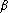pour que $\left(1\right)$ soit exacte sur ${𝒫}_{2}$.
2. En dťduire les valeurs de $k,\phantom{\rule{thickmathspace}{0ex}}\alpha$ etpour que $\left(1\right)$ soit d'ordre le plus ťlevť possible.
1. Calculer le noyau de Peano dans le cas oý $\left(1\right)$ est d'ordre $3$ et vťrifier que ce noyau est une fonction paire.
2. En dťduire qu'il existe $\xi \in \right]-1,\phantom{\rule{thickmathspace}{0ex}}1\left[$ tel que $E\left(f\right)=\frac{1}{135}{f}^{\left(4\right)}\left(\xi \right)$$E\left(f\right)$ est l'erreur d'intťgration.
3. Donner la formule d'intťgration relative ŗ $\left(1\right)$ sur $\left[a,\phantom{\rule{thickmathspace}{0ex}}b\right].$
4. Estimer l'erreur d'intťgration obtenue par la mťthode composťe associťe ŗ $\left(1\right)$ sur $\left[a,\phantom{\rule{thickmathspace}{0ex}}b\right]$ avec un pas constant $h=\frac{b-a}{n}$.

Exercice
On considťre la formule d'intťgration suivante :
${\begin{array}{c}\int \end{array}}_{0}^{\pi }f\left(x\right)\mathrm{sin}\left(2x\right)\phantom{\rule{thickmathspace}{0ex}}\mathrm{dx}\approx {a}_{1}f\left({x}_{1}\right)+{a}_{2}f\left({x}_{2}\right)$
1. Dťterminer ${a}_{1},\phantom{\rule{thickmathspace}{0ex}}{a}_{2},\phantom{\rule{thickmathspace}{0ex}}{x}_{1}$ et ${x}_{2}$ de sorte que cette formule soit exacte sur ${𝒫}_{3}$.
2. Calculer alors l'ordre de cette mťthode.

Exercice
1. Soit $f\left(x\right)=\frac{1}{1+{x}^{2}}$. Montrer qu'il existe un polynŰme $P$ unique de degrť $2$ vťrifiant:
$P\left(0\right)=f\left(0\right),\phantom{\rule{thickmathspace}{0ex}}P\left(1\right)=f\left(1\right)\phantom{\rule{thickmathspace}{0ex}}\text{et}P\left(\frac{1}{2}\right)=f\left(\frac{1}{2}\right).$
Dťterminer ${\begin{array}{c}\int \end{array}}_{0}^{1}P\left(t\right)\mathrm{dt}$ et la comparer ŗ ${\begin{array}{c}\int \end{array}}_{0}^{1}f\left(t\right)\mathrm{dt}$.
2. On considťre ${\begin{array}{c}\int \end{array}}_{0}^{1}\mathrm{sin}\frac{\pi {t}^{2}}{2}\mathrm{dt}$. On veut calculer cette intťgrale avec une erreur infťrieur ŗ ${10}^{-3}$.
1. Dťterminer le pas $h$ nťcessaire pour la mťthode des trapŤzes.
2. Dťterminer le pas $h$ nťcessaire pour la mťthode de Simpson.

Exercice
1. Trouver l'ordre des formule de : rectangle, trapŤze et Simpson.
2. Pour $f\in {𝒞}^{4}\left(\left[-1,1\right]\right)$. On pose
$E\left(f\right)={\begin{array}{c}\int \end{array}}_{-1}^{1}f\left(x\right)\phantom{\rule{thickmathspace}{0ex}}\mathrm{dx}-\frac{2}{6}\left[f\left(-1\right)+4f\left(0\right)+f\left(1\right)\right]\phantom{\rule{2em}{0ex}}hbox\mathrm{et}\phantom{\rule{2em}{0ex}}{K}_{3}\left(t\right)=E\left(x⟶\left(x-t{\right)}_{+}^{3}\right)$
Montrer que ${K}_{3}\left(t\right)={\begin{array}{c}\int \end{array}}_{t}^{1}\left(x-t{\right)}^{3}\phantom{\rule{thickmathspace}{0ex}}\mathrm{dx}-2\left(\frac{2}{3}{t}_{-}^{3}+\frac{1}{6}\left(1-t{\right)}^{3}\right)$.
3. En dťduire ${K}_{3}\left(t\right)=-\frac{1}{12}\left(1-\mid t\mid {\right)}^{3}\left(1+\mid t\mid \right)$. Calculer par deux mťthodes diffťrentes ${\begin{array}{c}\int \end{array}}_{-1}^{1}{K}_{3}\left(t\right)\mathrm{dt}$.
4. Enoncer le thťrŤme de Peano et montrer que si $f\in {𝒞}^{4}\left(\left[a,b\right]\right)$ alors on a :
$\mid {\begin{array}{c}\int \end{array}}_{\mathrm{af}}^{b}\left(t\right)\mathrm{dt}-\frac{h}{6}\left(f\left(a\right)+2\sum _{k=1}^{n-1}f\left({a}_{k}\right)+4\sum _{k=0}^{n-1}f\left({a}_{k}+\frac{b-a}{2n}\right)+f\left(b\right)\right)\mid \le$
$\frac{\left(b-a{\right)}^{5}}{2880{n}^{4}}\underset{\left[a,b\right]}{\mathrm{sup}}\mid {f}^{4}\left(t\right)\mid$

document sur quelques mťthodes d'intťgration numťrique (niveau licence).
: numerical_method,integral, interactive mathematics, interactive math, server side interactivity